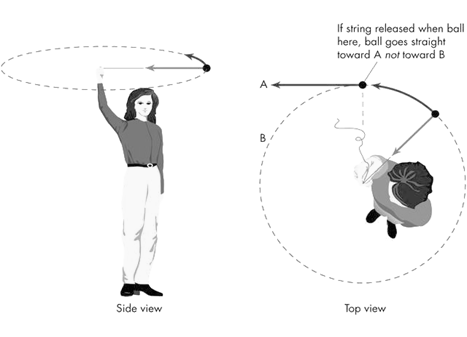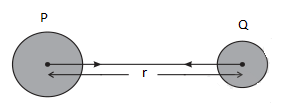# Chapter 10 Gravitation Notes (Physics)

Welcome to Class 9 Gravitation Notes for Chapter 10.The topics in this page are What Is Gravitation ,Universal law of gravitation and Free Fall.This is according to CBSE and the NCERT textbook. If you like the study material, feel free to share the link as much as possible.

Table of content

## What Is Gravitation

• In our dairy life we have noticed things falling freely downwards towards earth when thrown upwards or dropped from some height.
• Fact that all bodies irrespective of their masses are accelerated towards the earth with a constant acceleration was first recognized by Galileo (1564-1642).
• The motion of celestial bodies such as moon, earth, planets etc. and attraction of moon towards earth and earth towards sun is an interesting subject of study since long time.
• Now few question arises that are
(a) what is the force that produces such acceleration with which earth attract all bodies towards the center. Learn more about class 9 force and laws of motion notes
(b) What is the law governing this force.
(c) Is this law is same for both earthly and celestial bodies.
• Answer to this question was given by Newton as he declared that
Laws of nature are same for earthly and celestial Bodies.
The force between any object falling freely towards earth and that between earth and moon are governed by the same laws.
• Kepler (1571-1631) Studied the planetary motion in detail and formulated his three laws of planetary motion, which were available Universal law of gravitation.

• You must have observed that whenever you throw any object upwards it reaches a certain height and then falls downward towards the Earth. So these objects are acting under the gravitational pull of the Earth or gravitational forces which are forces of attraction.
• Gravitational force or gravity of earth is responsible for pulling you and keeping you on earth.
• Now each and every object in this universe that has mass exerts a gravitational force on every other mass and the size of that pull depends on how large or small are the masses of two objects under consideration.
• So for smaller masses like two human beings the gravitational force of attraction is very small and is negligible because two peoples are not very massive
• Now when you consider massive objects like planets, Sun, Earth, Moon or other celestial bodies, the gravitational pull becomes very strong.
• So here you must note that gravitational force depends on how massive objects under consideration are.
• Gravity is very important on earth . It is the gravitational pull of earth that keeps our planet orbiting round Sun.
• The motion of moon is also affected by both Sun and Earth.

### Why don't Moon Fall down

• You must wonder If gravitational force is a force of attraction then why does moon not fall into earth?
• To understand this consider a person whirling a stone tied to a thread along a circular path as shown below in the figure.• If he releases the stone then it flies along the tangent , at that point on the circular path.
• Before the release of thread it is centripetal force responsible for the motion of stone in the circular path where the stone moves with a certain speed and changes direction at every point.
• During this motion the change in direction involves change in velocity which produces acceleration. This force which is called centripetal force , causes this acceleration and keeps the body moving along the circular path is acting towards the center.
• Now when the thread is released the stone does not experience this force and flies off along a straight line that is tangent to the circular path.
• The motion of the moon around the earth is due to the centripetal force. The centripetal force is provided by the force of attraction of the earth. If there were no such force, the moon would pursue a uniform straight line motion.

## Universal Law of Gravitation

• Universal Law of gravitation was formulated by Sit Issac Newton.
• It states that
Every body in universe attracts every other body with a force which is directly proportional to the product of their masses and inversely proportional to the square of distance between them. The force acts along the line joining the two bodies
• To understand that let us consider two objects of masses $M$ and $m$ that lie at a distance $r$ from each other as shown below in the figure• Let $F$ be the force of attraction between two objects $P$ and $Q$.
• Now according to the universal law of gravitation as stated earlier , this force $F$ is proportional to the product of masses of objects $P$ and $Q$ that is
$F \propto M \times m$              (1)
and the force is inversely proportional to the square of distance between these two objects that is
$F \propto \frac{1}{{{r^2}}}$             (2)
• Combining both equations (1) and (2) we get
$F \propto \frac{{Mm}}{{{r^2}}}$             (3)
or
$F = G\frac{{Mm}}{{{r^2}}}$             (4)
Here G is the constant known as Universal Gravitational constant.
• SI unit of gravitational constant G is $N{m^2}K{g^{ - 2}}$
• Value of G was first found by Henry Cavendish (1731-1810) and it is
$G = 6.673 \times {10^{ - 11}}N{m^2}K{g^{ - 2}}$

### Important Characteristics of Gravitational forces

• The gravitational force is a central force that is It acts along the line joining the centers of two bodies.
• it is a long range force. The gravitational force is effective even at large distances.
• It is a conservative force. This means that the work done by the gravitational force in displacing a body from one point to another is only dependent on the initial and final positions of the body and is independent of the path followed.
• Unlike electrostatic and magnetic forces, the gravitational force is always attractive.

### Why is this Universal Law of Gravitation so important

• It is the force that is responsible for binding us to Earth.
• It is responsible for the motion of moon around the earth
• It is responsible for the motion of planets around the sun
• The tides formed by rising and falling of water level in the ocean are due to the gravitational force exerted by both sunand moon on the earth.

## Free Fall

• Due to gravitational force Earth attracts every object towards it.
• So whenever an object falls towards earth it falls under the influence of gravitational force and if only gravitational force acts on the object during its free fall then such a motion of object is said to be free fall motion
The falling of a body (or object) from a height towards the earth under the gravitational force of earth (with on other force acting on it) is called free fall
• When an object falls freely towards the earth there happens to be no change in the direction of motion of the object but due to the attraction of earth there will be a change in the magnitude of velocity.
• Change in velocity involves acceleration. So whenever the objects falls towards earth an acceleration is involved due to earth's gravitational force.
• This acceleration due to earth's gravitational force is called acceleration due to gravity which is denoted by 'g' and its unit is m/s2

### Calculation of value of g

• We shall now find the acceleration due to gravity 'g'
• Let us consider an object of mass $m$ falling freely under the influence of Earth's gravitational force.
• Since the object is moving under the sole effect of gravity (free fall motion) then acceleration experienced by the object would be g which is the acceleration due to gravity of earth.
• Now from Newton's second law of motion , force experienced by this freely falling object would be
$F_{grav}=mg$                 (5)
• Again from Universal law of gravitation force of gravity with which an object is attracted to earth is
${F_{grav}} = G\frac{{{M_{earth}} \times m}}{{{d^2}}}$              (6)
where $d$ is the distance from the center of the object to the center of the earth and $M_{earth}$ is the mass of the earth.
• Now from equations (5) and(6) we have $mg = G\frac{{{M_{earth}} \times m}}{{{d^2}}}$
or, $g = G\frac{{{M_{earth}}}}{{{d^2}}}$                (7)
• So if the object under consideration is very near to the surface of the earth or on the surface of the earth then we can approximate $d \approx {R_{earth}}$ where $R_{earth}$ is the radius of the earth. So expression for g near or on the surface of the earth would be
$g = G\frac{{{M_{earth}}}}{{{{({R_{earth}})}^2}}}$                (8)
• One more important point here to note is that earth is not a perfect sphere. As the radius of earth increases from the poles to the equator , the value of g becomes greater at the poles then at the equator
• Now to calculate the value of g we shall put the values $G = 6.7 \times {10^{ - 11}}N{m^2}K{g^{ - 2}}$
${R_{earth}} = 6.4 \times {10^6}m$
${M_{earth}} = 6 \times {10^{24}}Kg$
So,
$g = G\frac{{{M_{earth}}}}{{{{({R_{earth}})}^2}}}$
putting in above values and calculating we find
$g = 9.8m{s^{ - 2}}$

### motion of objects under the influence of Earth's gravity

• From equation (8) we can see that the acceleration that object experiences during the free fall is independent of the mass of the object undergoing motion
• This implies that both light or heavy, small or big, hollow or solid objects would fall at the same rate.
• As the value of acceleration due to gravity is constant near earth , we can now write the equations of motion that we studied in class 9 motion notes would be valid for free fall motion if we replace acceleration 'a' with acceleration due to gravity 'g'
• So in this case equations of motion would be
$v=u+gt$     when object is falling downwards
$v=u-gt$     when object is thrown upwards
$h=u+\frac{1}{2}gt^{2}$
abd $v^{2}-u^{2}=2gh$
where $h$ is the height of the object from the ground.

## Summary

Here is the Class 9 Gravitation notes Summary
• According to the law of gravity, the force of attraction between any two objects is inversely proportional to the square of their distance and directly related to the product of their masses.
• Gravity is the name for the earth's gravitational force.Unless there are massive masses involved, gravity is a weak force.
• The weight is determined by multiplying the mass by the gravitational acceleration.